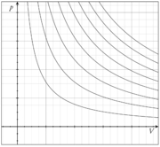xIdeal gas law
Posts  1 - 1  of  1EOSseeker
What is the density of a mixture of CO2 and N2 gases in mol/m3 by Peng-Robinson equation of state?
T=225K and Pressure= 68.759 MPa and Z=1.67661.
(Mole fraction of Co2= 10.56% and mole fraction of N2 is 89.44%)

Critical temperature (Tc) for CO2 = 304.19 K ,
Critical pressure (Pc) for CO2 = 73.825 bar , 72.85 atm , 1070.67 psia
Acentric factor (ω) for CO2 = 0.228

Critical temperature for N2 = 126.1 K
Critical pressure for N2 = 33.999 bar , 33.5 atm , 492.26 psia
Acentric factor for N2 = 0.040

This is Peng–Robinson equation of stat:

P=(RT/V-b)-(θ/(V(V+b)+b(V-b))

a=(0.45724(R^2)(Tc^2))/Pc

b=(0.0778(R)(Tc))/Pc

θ = a[1+(0.3764+1.5422ω-0.26992ω^2 )(1-(T/Tc )^0.5 )]^2

ω is the acentric factor.